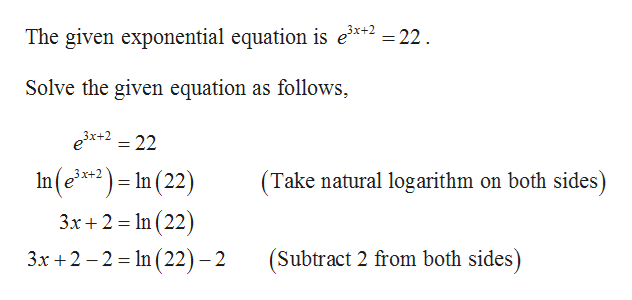# For Exercise, solve the equation or inequality. Write the solution set to the inequalities in interval notation if possible.e 3x+2 = 22

Question
1 views

For Exercise, solve the equation or inequality. Write the solution set to the inequalities in interval notation if possible.

3x+2 = 22

check_circle

Step 1help_outlineImage TranscriptioncloseThe given exponential equation is e*2 = 22. Solve the given equation as follows, ex+2 = 22 In(e**) = In (22) 3x+2 (Take natural logarithm on both sides) %3D 3x + 2 = In (22) 3x +2 – 2 = In (22)- 2 %3D (Subtract 2 from both sides) fullscreen

### Want to see the full answer?

See Solution

#### Want to see this answer and more?

Solutions are written by subject experts who are available 24/7. Questions are typically answered within 1 hour.*

See Solution
*Response times may vary by subject and question.
Tagged in

### Other